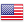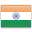Grade "A+" Accredited by NAAC with a CGPA of 3.46
Grade "A+" Accredited by NAAC with a CGPA of 3.46

Course ID
FC 202
Level
Program
BBA (FIA)
Semester
Second
Credits
6.0
Paper Type
Core Course
Method
Lecture & Tutorial

## Unique Paper Code: 61011201

To familiarize the students with various Statistical Data Analysis tools that can be used for effective decision making. Emphasis will be on the application of the concepts learnt to various managerial situations.

## Learning Outcomes:

At the end of the course, students should be able to:

• Summarize data sets using Descriptive statistics
• Analyze the relationship between two variables of various managerial situations
• Geometrically Interpret Correlation and Regression
• Develop managerial decision problems using Probability Density Functions and Cumulative Density Functions

## Course Contents

Unit I
Unit II
Unit III
Unit IV

Unit I (2 Weeks)

Data: quantitative and qualitative, attributes, variables, Scales of measurement: nominal, ordinal, interval and ratio, Measures of Central Value: Meaning, Need for measuring central value. Characteristics of an ideal measure of central value. Types of averages – mean, median, mode, harmonic mean and geometric mean. Merits, Limitations and Suitability of averages. Relationship between averages. Measures of Dispersion: Meaning and Significance. Absolute and Relative measures of dispersion – Range, Quartile Deviation, Mean Deviation, Standard Deviation, Moments, Skewness, Kurtosis, z-score, Chebyshev and empirical rule. Graphical Analysis of Ordered Data: Histograms, Stem and Leaf Plots, Box-and-Whisker Plots

References:

S.P. Gupta, Statistical Methods, Sultan Chand & Sons [Vol I, Chapter 5,6,7,8 and 9]

Kellar, Statistics for Management, Cengage Learning [Chapter 2, 3 and 4]

Unit II (4 Weeks)

Correlation Analysis: Meaning and significance. Correlation and Causation, Types of correlation, Methods of studying simple correlation – Scatter diagram, Karl Pearson’s coefficient of correlation, Spearman’s Rank correlation coefficient.

Regression Analysis: Meaning and significance, Regression vs. Correlation, Simple Regression model: Linear Regression, Conditions for simple linear regression, Standard error of estimate, Geometric Interpretation of Regression.

References:

S.P. Gupta, Statistical Methods, Sultan Chand & Sons [Vol I, Chapter 10, 11]

Unit III (3 Weeks)

Probability: Meaning and need, Theorems of addition and multiplication, Conditional probability, Bayes’ theorem, Random Variable- discrete and continuous. Probability Distribution: Meaning, characteristics (Expectation and variance) of Binomial, Poisson, exponential and Normal distribution, Central limit theorem.

References:

S.P. Gupta, Statistical Methods, Sultan Chand & Sons [Vol II, Chapter 1, 2]

Levin and Rubin, Statistics for Management, Pearson. [Chapter 4, 5]

Unit IV (3 Weeks)

Estimation of population mean, Confidence intervals for the parameters of a normal distribution (one sample only), Introduction to testing of Hypothesis: Concept; Level of Significance; Process of testing; Type I and Type II error, Test of hypothesis concerning Mean: z test & t test (One Sample), Proportion Z test, Non parametric tests: One-Sample Wilcoxon Signed Rank Test, Paired-Sample Wilcoxon Signed Rank Test, Kolmogorov-Smirnov Test.

References:

Levin and Rubin, Statistics for Management, Pearson. [Chapter 7, 8, 14]

Kellar, Statistics for Management, Cengage Learning [Chapter 10, 11, 12 19]

#### Text Books

Gupta S.P. (2017) : Statistical Methods, Sultan Chand & Sons, 45h Revised Edition
Levin, R. and Rubin, D. (2017). Statistics for Management. 8thed. New Delhi: Pearson.
Keller, G. (2009). Statistics for Management, Indian Edition, New Delhi: Cengage Learning.

Stine, R. and Foster. (2014). Statistics for Business (Decision making and Analysis). 2nded. New Delhi: Pearson
Vohra, N.D. (latest edition) Business Statistics, New Delhi, Mcgraw Hills

#### Teaching Learning Process

The teaching learning process will consist of imparting key concepts of statistics to the students. To make the lectures interesting use of PPTs and audio visual presentation is advisable as and when needed. The students should be encouraged to solve real life business/ managerial problems through software like MS Excel

#### Assessment Methods

1. Internal Assessment: 25 marks
2. Written Theory Exam: 75 marks
Assessment of the students will take place on a continuous evaluation basis. It will primarily consist of class test, assignment, presentation and attendance.

#### Keywords

Descriptive Statistics, data handling, Correlation and Regression, Probability, Testing of hypothesis.

Disclaimer: Details on this page are subject to change as per University of Delhi guidelines. For latest update in this regard please refer to the University of Delhi website here.Englishहिन्दी